# Boxfill Tutorial¶

This is an in-depth introduction to the boxfill graphics method in VCS. It breaks down each of the important attributes of the graphics method, how to use them, and what effects they have on your plots.

The CDAT software was developed by LLNL. This tutorial was written by Charles Doutriaux. This work was performed under the auspices of the U.S. Department of Energy by Lawrence Livermore National Laboratory under Contract DE-AC52-07NA27344.

## Setup¶

This is just the normal setup for a plot in VCS; we get the data, retrieve a variable, initialize our canvas, and plot a basic boxfill.

In :
import vcs, cdms2
import os
if not os.path.exists(vcs.sample_data):
f = cdms2.open(os.path.join(vcs.sample_data, "clt.nc"))
s = f('clt')
x = vcs.init(bg=True)
box = vcs.createboxfill()
x.plot(s, box)

Out: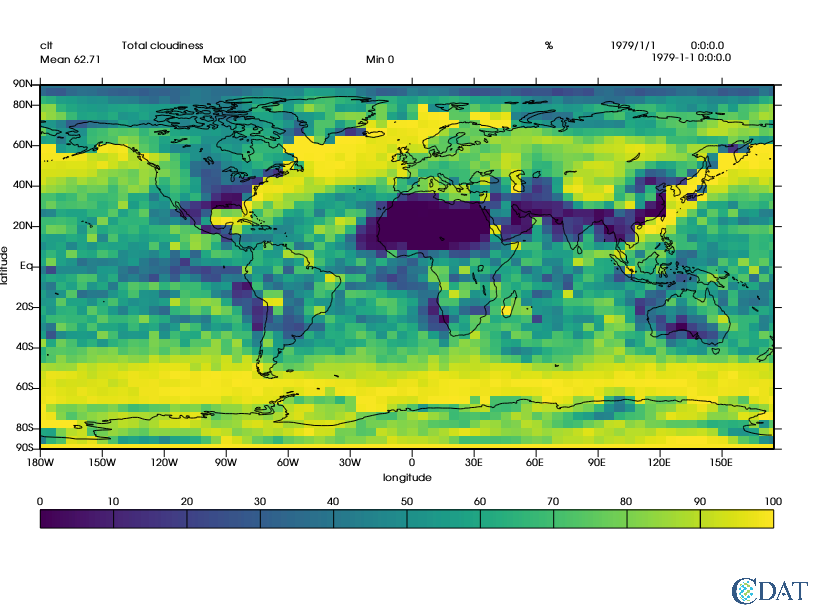#### Tip 1:¶

You can use the list() function on most VCS objects to see what their attributes are

In :
box.list()

---------- Boxfill (Gfb) member (attribute) listings ----------
graphics method = Gfb
name = __boxfill_470823546068748
projection = linear
xticlabels1 = *
xticlabels2 = *
xmtics1 =
xmtics2 =
yticlabels1 = *
yticlabels2 = *
ymtics1 =
ymtics2 =
datawc_x1 = 1e+20
datawc_y1 =  1e+20
datawc_x2 =  1e+20
datawc_y2 =  1e+20
datawc_timeunits =  days since 2000
datawc_calendar =  135441
xaxisconvert =  linear
yaxisconvert =  linear
boxfill_type =  linear
level_1 =  1e+20
level_2 =  1e+20
levels =  [1e+20, 1e+20]
color_1 =  0
color_2 =  255
fillareacolors =  None
fillareastyle =  solid
fillareaindices =  
fillareaopacity =  []
fillareapixelspacing =  None
fillareapixelscale =  None
legend =  None
ext_1 =  False
ext_2 =  False
missing =  (0.0, 0.0, 0.0, 100.0)


## The Attributes¶

So, let's begin working our way through the attributes in the list() output.

### box.projection¶

Projections change the mappings of spherical coordinates (longitude and latitude) to cartesian coordinates (X/Y). The "linear" projection maps each degree of lon/lat to an X/Y point directly, so each degree is equidistant, but that does cause some distortion of landmasses/data in parts of the globe. To get a good look at the north/south pole, a better projection to use is the "polar" projection. Each projection can be customized to get the exact mapping you want, but that's a subject for a different tutorial.

In :
box.projection = "polar"
x.clear()
x.plot(s, box)

Out: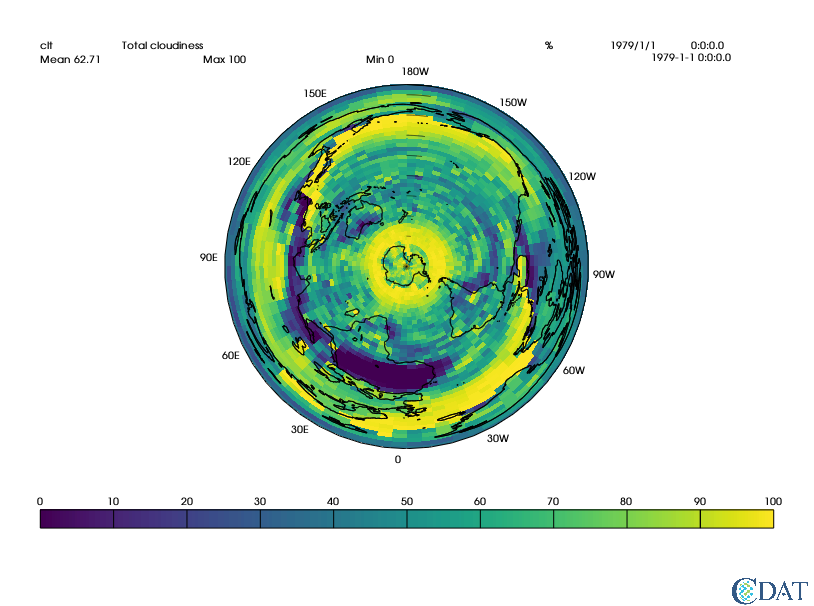### box.(x|y)ticlabels(1|2)¶

These attributes control where tick marks appear on the axes of the plot. You pass in a dictionary that maps axis values (lon/lat) to string labels.

Note: You can also set ticlabels to the name of a vcs "List", which are a series of predefined mappings that you can look at using vcs.listelements("list")

VCS will determine the correct position (in X/Y) for your axis label and tick mark to appear, and place them there. In the below example, we'll set the positions for a few nice landmarks in lon/lat, and be able to see their locations on the map. xticlabels1 controls tick marks on the bottom X axis; xticlabels2 controls the marks on the top of the axis. yticlabels1 controls the left Y axis, and yticlabels2 controls the right Y axis.

#### Tip 2:¶

xticlabels2 and yticlabels2 don't display labels when using the default template in VCS. To make them appear, you have to create a new template (vcs.createtemplate()) and set the xlabel2 and/or ylabel2 priority to >0 (xlabel2.priority = 1). Priority of 0 on a template attribute prevents that attribute from being displayed; a handy way to hide any element of the plot that you don't want displayed!

In :
box.projection = "linear"
box.xticlabels1 = {0: "Prime Meridian", -121.7680: "Livermore", 37.6173: "Moscow"}
box.yticlabels1 = {0: "Eq.", 37.6819: "L", 55.7558: "M"}
x.clear()
x.plot(s, box)

Out: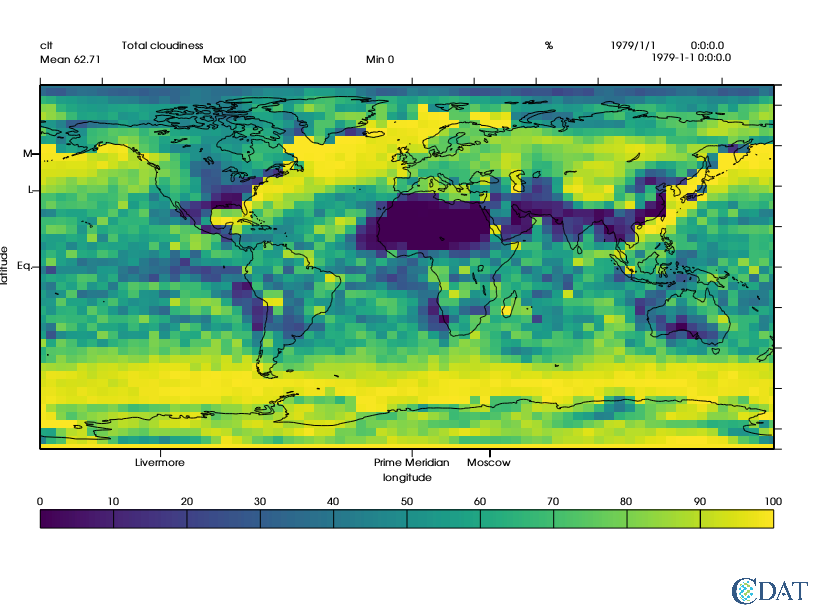### box.(x|y)mtics(1|2)¶

The mtics are "mini" ticks; they are meant to be smaller, intermediate tickmarks without labels that show up between the larger ticks. The default template does not display them; you have to use a custom template and set their priority to 1. Once again, xmtics1 points to the bottom, xmtics2 the top, ymtics1 the left, and ymtics2 the right. Below, we use the names of some of the predefined VCS lists that were mentioned in the previous section.

In :
box.xmtics1 = "lon30"  # Every 30deg
box.ymtics1 = "lat20"  # Every 20deg
template = vcs.createtemplate()
template.xmintic1.priority = 1
template.ymintic1.priority = 1
x.clear()
x.plot(s, box, template)

Out: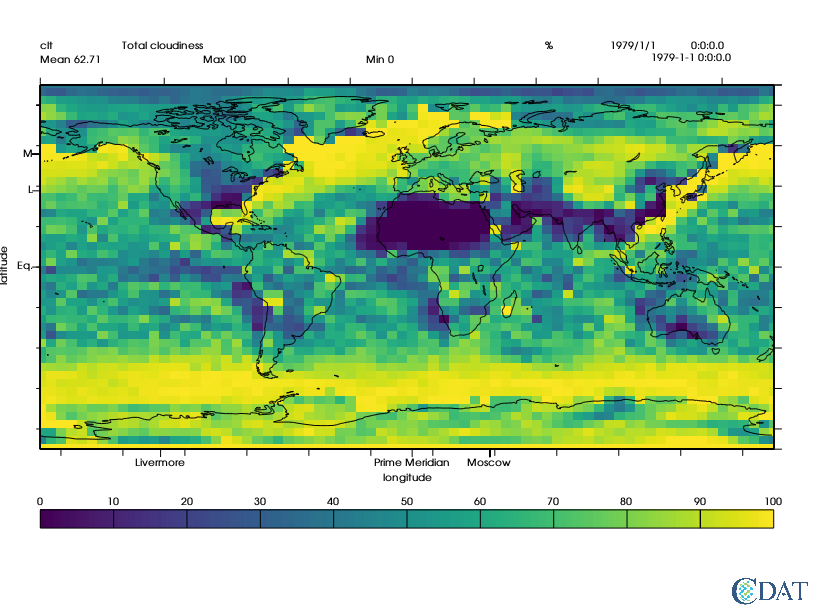### box.datawc_(x|y)(1|2)¶

The datawc_* attributes control the "world coordinates" of the plot. That is, they describe the section of the data to display in the plot. You specify values in the coordinate space of the axes of your data; for most of us, that means lon/lat values. This then allows you to reduce the displayed portion of your data to a specific area. Here, we'll zoom in on Livermore, and use the mini ticks to show the exact point that Livermore is located at. X1 is the start of the x axis, X2 is the end of the x axis; the same applies to Y1 and Y2.

In :
box.datawc_x1 = -125
box.datawc_x2 = -117
box.datawc_y1 = 34
box.datawc_y2 = 38
box.xmtics1 = box.xticlabels1
box.ymtics1 = box.yticlabels1
box.xticlabels1 = "*"
box.yticlabels1 = "*"
save_xmin_y1 = template.xmintic1.y1
save_xmin_y2 = template.xmintic1.y2
save_ymin_x1 = template.ymintic1.x1
save_ymin_x2 = template.ymintic1.x2
template.xmintic1.y1 = template.data.y1
template.xmintic1.y2 = template.data.y2
template.ymintic1.x1 = template.data.x1
template.ymintic1.x2 = template.data.x2
x.clear()
x.plot(s, box, template, ratio="autot")

Out: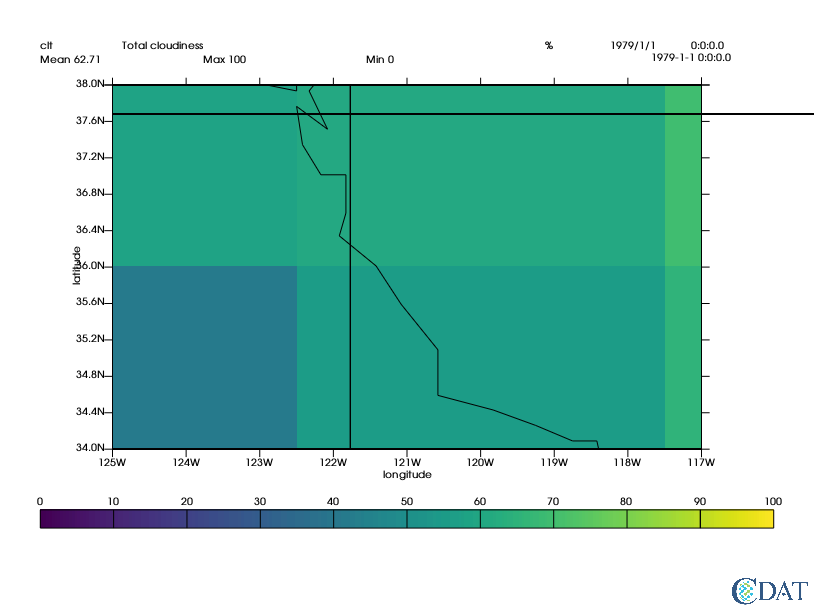#### Tip 3:¶

1e20 is the "automatic" value for datawc_* attributes, and uses the entire dataset as provided to the plot call.

### box.level_(1|2)¶

level_1 and level_2 control what the minimum and maximum values of data displayed in the plot will be. They allow you to specify the exact range of values to use in the legend of the plot. Values outside of those ranges will be left blank on the plot. To reset them, set them to 1e20.

In :
box.datawc_x1 = 1e20
box.datawc_x2 = 1e20
box.datawc_y1 = 1e20
box.datawc_y2 = 1e20
box.xmtics1 = None
box.ymtics1 = None
box.level_1 = 20
box.level_2 = 40
x.clear()
x.plot(s, box, template)

Out: